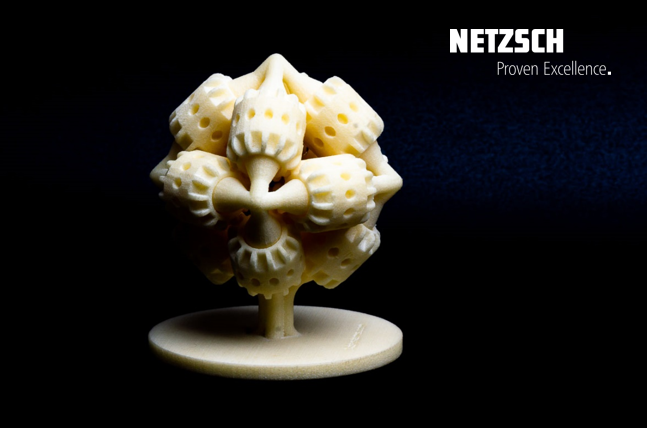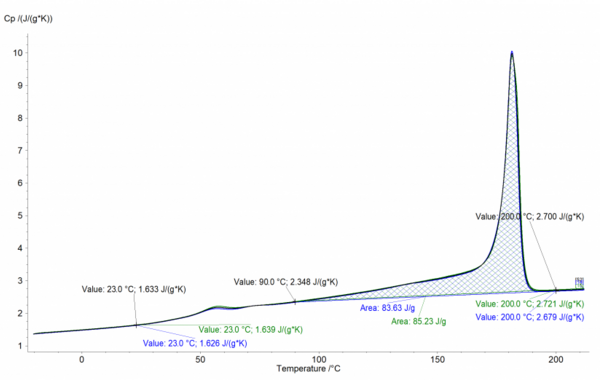06.04.2021 von Dr. Natalie Rudolph, Dr. Stefan Schmölzer

# Measuring Specific Heat Capacity to Simulate SLS Processes

Significant efforts have been made to model and simulate the Selective Laser Sintering process as information about the temperature field in lower layers is difficult to measure. Learn how specific heat capacity can help!

During the SLS (Selective Laser Sintering) process, the previously melted layers disappear into the powder bed surrounded by unsintered powder. Therefore, information about the temperature field in lower layers is difficult to measure. Thus, significant efforts have been made to model and simulate the SLS process. Two quantities that are essential for this are the specific heat capacity (cp) and thermal conductivity (k) as a function of temperature. When fillers are added, they do not only change the crystallization rate, but also require a higher build temperature due to the change in cp and k.

#### How to determine the specific heat capacity

To determine the specific heat capacity as a function of temperature of different materials, Differential Scanning Calorimetry (DSC) is used. The heat capacity is defined as the quantity of heat necessary to raise the temperature of 1 g substance by 1°C while the pressure p is kept constant. It is described by the heat conduction equation:

In accordance with DIN EN ISO 11357-4 (and ASTM E1269), the sample is measured relative to a second (reference) sample of known heat capacity. A typical reference sample would be sapphire. Therefore, one experiment consists of three different runs in the temperature range of interest. The first is a scan with two empty pans (baseline), the second a scan with one pan containing the sapphire sample (reference), and finally the third run with the actual sample (sample) in the same type of pan.

Cp as a function of temperature of the sample can be calculated as follows:

#### Performing cp measurements on SLS polymer powder samples

In this example of a polymer powder, more specifically PA12, the measurements were performed using a NETZSCH DSC 204 F1 Phoenix® in accordance with the standard. After an initial cooling step to -25°C, the temperature was increased to 215°C at 10 K/min. Two different samples were measured and the average was calculated. All measurement conditions are summarized in the following table:

Table 1: Measurement conditions

The analysis in the NETZSCH Proteus® software is shown in Figure 1. It shows the „apparent“ specific heat capacity, superimposed with by the melting peak and glass transition.Figure 1: Specific heat capacity, Spezifische Wärmekapazität (cp)Die spezifische Wärmekapazität oder Wärmekapazität ist eine messbare physikalische Größe, die dem Verhältnis der einem Objekt zugeführten Wärme zur resultierenden Temperaturänderung entspricht.cp, obtained from two repetitive measurements (green and blue lines) as well as the calculated average (black line)

The cp data can be easily deduced from this curve. However, in the temperature range between 90-190°C, the effect of the increasing cp and the endothermic effect of melting are opposing each other. Therefore, the values in the melting range are typically interpolated. In the case of PA12 shown here, the interpolation would be performed between 90°C (2.348 J/gK) and 200°C (2.7 J/gK), which are indicated in the graph. The values can then be exported for subsequent use in material and process simulations, e.g., for the temperature filed and solidification in the SLS process. Another application of the data is the calculation of the thermal conductivity from thermal diffusivity and density data.